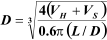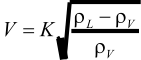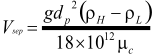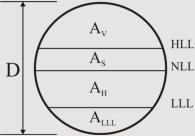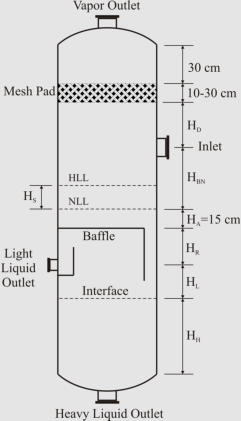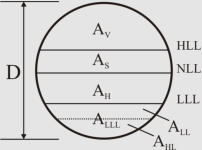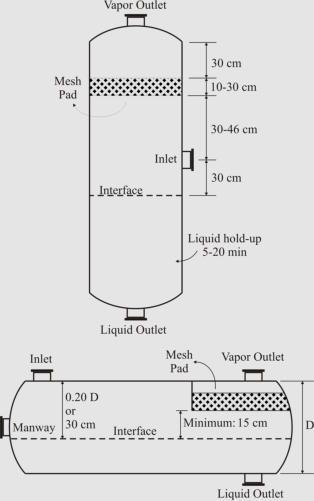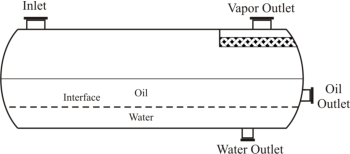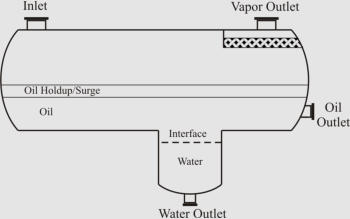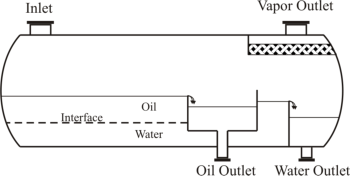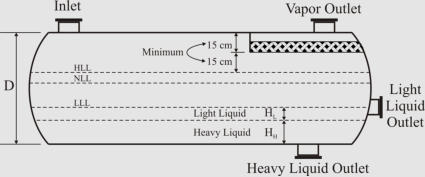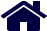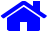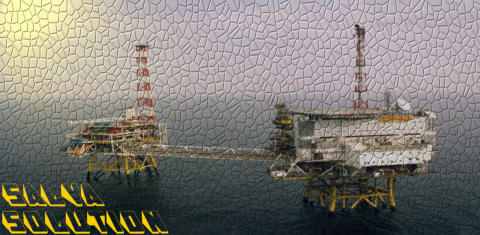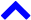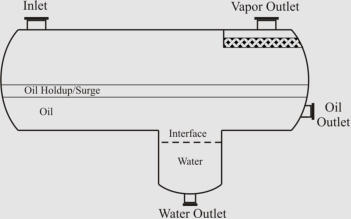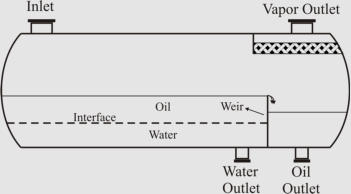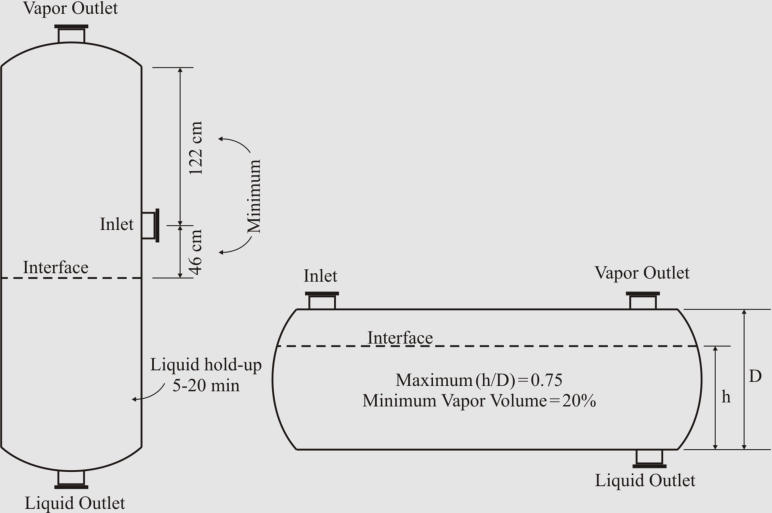Separator Sizing Here we review important guidelines and procedures for sizing multiphase separators: General Guidelines Droplet Settling Theory Liquid Retention Time Sizing Procedure Improvements Offered by CFD Simulations General Guidelines Orientation For    practical    design    of    separators,    it    is    necessary    to    compare    both    horizontal    and    vertical orientation   designs   to   determine   which   design   is   more   economical   (Svrcek   and   Monnery,   1993). However,    the    following    general    guidelines    are    recommended    to    be    considered    while    sizing multiphase separators. Vertical orientation advantages : Occupy little plot space Easier than horizontal separators to transport and install Used   when   there   is   small   liquid   load,   limited   plot   space,   or   where   ease   of   level   control   is desired (Evans, 1974) Preferred for operating either low or very high gas/oil ratio fluids (Arnold and Stewart, 2008) Horizontal orientation advantages : Higher operating capacity, and processing capabilities Preferred   when   emulsions,   foam,   or   high   gas/oil   ratio   fluids   are   present   (Arnold   and   Stewart, 2008) Separator Aspect Ratio (Length/Diameter) Plot restrictions and economic measures specify the separator aspect ratio. Different ranges have been suggested as shown in the table below Economic   studies   provided   the   separator   aspect   ratio   as   a   function   of   the   operating   pressure (Walas, 1990; Svrcek and Monnery, 1993). The recommended values presented in the table . The   figures   below   show   illustrated   guidelines   proposed   by   Walas   (1990)   and   Watkins   (1967)   for two-phase separators with and without demisters, respectively. Design Heuristics Proposed by Walas (1990) for Two-Phase Separators Equipped with Wire Mesh Demisters. Design Heuristics Proposed by Watkins (1967) for Two-Phase Separators. There   are   also   some   general   guidelines   for   choosing   among   different   horizontal   separators   (see the   figure   below)   which   are   worth   mentioning:   As   per   Arnold   and   Stewart   (2008),   while   the simple   design   is   easily   adjustable   to   handle   unexpected   changes   in   liquid   density/flow   rates,   the bucket   &   weir   design   is   used   when   interface   level   control   is   difficult   (emulsions   or   paraffin problems).   If   heavy   liquid   mass   fraction   is   substantial,   the   weir   design   is   preferable,   but   the   boot design is preferred when heavy liquid mass fraction is less than 20% (Monnery and Svrcek, 1994).                                                                                               ( a )                                                                                                                                                                                                                                           ( b)                                            ( c)                                                                                        ( d) Different Common Designs of Horizontal Three-Phase Separators; (a) “Simple”, (b) “Boot”, (c) “Weir”, and (d) “Bucket and Weir”. Droplet Settling Theory Liquid Droplet in Vapor Phase The   force   balance   between   drag   and   gravitational   forces   exerted   on   a   liquid   droplet   leads   to   its terminal settling velocity : Drag    coefficient    for    vapor-liquid    separation    can    be    obtained    from    the    diagram    proposed    by GPSA.   This   diagram   is   applicable   if   Re p    is   between   0.4   and   1500.   The   diagram   curve   can   be regressed as shown in the following with SI units (Monnery and Svrcek, 2000) : As   presented   in   the   table,   there   are   different   values   recommended   by   the   field   researchers   for   the design droplet size . In    separator    sizing    literature,    it    is    very    common    to    rearrange    the    settling    velocity    equation introducing   a   K   coefficient,   known   as   the   settling   velocity   coefficient.   This   leads   to   the   Sauders- Brown equatio n: Different   values   are   suggested   in   the   literature   to   be   used   for   the   settling   velocity   coefficient.   The following table provides some of these suggestion s: More   sophisticated   approaches   recommend   that   pressure   functionality   need   be   considered   for   the settling   velocity   coefficient.   The   approaches   proposed   by   York   Mist   Eliminator   Company   and GPSA are given at below (Svrcek and Monnery, 1993): York Mist Eliminator Compan y GPS A Liquid Droplet in Liquid Phase Similar    to    vapor-liquid    separation,    the    force    balance    between    drag    and    gravitational    forces exerted on a liquid droplet leads to its terminal velocity It   is   generally   assumed   that   Re p    is   lower   than   0.1   and   Stokes’   law   can   be   used   for   calculating   the drag coefficient: C D  = 24/Re p   This assumption results in a very simple equation for the liquid droplet terminal velocit y: Some design droplet sizes recommended in the literature are shown in the tabl e. Liquid Retention Time In   order   to   handle   upstream   or   downstream   variations   and   establish   smooth   and   safe   operation   of downstream   facilities,   an   adequate   liquid   retention   time   need   be   assumed.   This   assumption   may be   based   on   experience,   scale   model   predictions,   or   field   data.   Liquid   retention   time   is   combined from   holdup   and   surge   time.   Holdup,   considered   for   smooth   and   safe   operation   of   downstream facilities,   is   defined   as   the   time   it   takes   to   reduce   the   liquid   level   from   normal   to   low   liquid   level by   closing   inlet   flow   while   maintaining   a   normal   outlet   flow.   Surge   time,   considered   for   handling upstream   or   downstream   variations,   is   defined   as   the   time   it   takes   to   increase   the   liquid   level from   normal   to   high   liquid   level   by   closing   outlet   flow   while   maintaining   a   normal   inlet   flow. Some   rules-of-thumb   have   been   suggested   at   literature   which   are   not   in   reasonable   agreement with each other as shown in the table . In   the   more   pragmatic   approach   presented   by   Svrcek   and   Monnery   in   1993   and   1994,   low   liquid level   is   first   set   based   on   the   operating   pressure   and   diameter   (typical:   6-12   in).   Then,   holdup (typical:   2-10   min)   and   surge   times   (typical:   1-5   min)   are   specified   based   on   the   service   and   quality of the personnel and instrumentation (with impact factor of 1.0 – 1.5 altogether). Sizing Procedure The     sizing     approach     outlined     here     is     proposed     by     Svrcek     and     Monnery     through     two internationally   acclaimed   papers   in   1993   and   1994.   It   is   an   algorithmic   method   for   designing   the most     economical     separator.     Among     the     classic     methods,     the     method     offers     the     most comprehensive   approach   while   benefiting   from   the   accepted   industrial   design   guidelines.   As explained    in    the    following,    height    adjustments    are    made    for    sizing    vertical    separators    and iterative calculations are performed for finding the most economical horizontal separator. Sizing Vertical Two-Phase Separat o r 1. Calculate the terminal settling velocity of liquid droplets. 2. Set the vapor velocity equal to 75% of the terminal settling velocity. 3. Calculate the vessel diameter based on the vapor volumetric flow-rate and the vapor velocity. 4. Obtain low liquid level height (typical: 6-12 in). 5. Calculate the height from LLL to NLL based on the required holdup time (typical: 2-10 min). 6. Calculate the height from NLL to HLL based on the surge time (typical: 1-5 min). 7. Calculate the height from HLL to the inlet nozzle centerline based on the inlet nozzle size which can be calculated as:  8. Assume the disengagement height (H D ) to be about 0.5D (Min: 42 in or 30 in with demister). 9. Calculate the vessel height. If the aspect ratio is not in the range of 1.5-6.0, increase the diameter (to decrease the aspect ratio) or increase vapor disengagement height (to increase the aspect ratio) and fix the problem. Sizing Horizontal Two-Phase Separat or In   horizontal   arrangement,   cross-sectional   area   of   the   vessel   is shared   by   vapor-liquid   disengagement   area   and   areas   for   liquid retention and low liquid level. Here is the iterative algorithmic method for sizing: 1. Calculate the terminal settling velocity of liquid droplets. Use 0.75U T as the settling velocity (25% contingency). 2. Calculate the holdup volume based on the required holdup time (typical: 2-10 min) and liquid volumetric flow rate. 3. Calculate the surge volume based on the surge time (typical: 1-5 min) and liquid volumetric flow rate. 4. Assume an aspect ratio (L/D) based on the operating pressure and calculate an initial diameter:  5. Obtain LLL (typical: 6-12 in) and calculate A LLL . 6. Set H V  to the larger of 0.2D or 1 ft (no demister), 2 ft (with demister). Calculate A V . 7. Calculate L to accommodate the liquid retention volume: L = (V H +V S )/(A T -A V -A LLL ) 8. Calculate the minimum length required for vapor-liquid separation: L min  = (Q V /A V )(H V /U T ) 9. If L < 0.8L min , increase H V  and go to step 7. Else if L < L min , set L = L min . Else if L > 1.2L min , decrease H V  (if acceptable), and go to step 7. Else (L > L min ), L is acceptable. 10. If L/D < 1.5, decrease D and go to step 5. Else if L/D > 6, increase D and go to step 5. 11. Calculate the approximate vessel weight based on the thickness and surface area of shell and heads. 12. In order to find the optimum case (the minimum weight), change the vessel diameter by 6 in increments and repeat the calculations from step 5 while keeping the aspect ratio in the range of 1.5 to 6.0. Sizing Vertical Three-Phase Separator 1. Calculate the terminal settling velocity of light liquid droplets. 2. Set the vapor velocity equal to 75% of the terminal settling velocity. 3. Calculate the vessel diameter based on the vapor volumetric flow-rate and the vapor velocity. 4. Calculate the separation velocities of both liquid phases through each other. 5. Set the thickness of liquid phases (HLL = HHL =  0.3 m as minimum), and calculate the separation times for the liquid droplets (t HL  and t LH ).  6. Calculate the cross-sectional areas of the liquid phases. Note, A H  is the same as the vessel cross- sectional area (A H  = A), but the area allotted to baffle plate down-comer should be subtracted from A to obtain A L . 7. Calculate the residence time of the light liquid:    t RL  = H L A L /Q LL . If t RL  < t HL  increase the vessel diameter so that t RL  = t HL . 8. Calculate the residence time of the heavy liquid: t RH  = H H A H /Q HL . If t RH  < t LH  increase the vessel diameter so that t RH  = t LH . 9. Calculate the height of light liquid phase above the outlet (H R ) based on the required holdup time (typical: 2-10 min) and liquid flow rate. 10. Calculate the surge height (H S ) based on the surge time (typical: 1-5 min) and liquid flow rate. 11. Set liquid level above baffle (H A  = 0.15 m minimum). 12. Calculate the height from NLL to the inlet nozzle centerline based on the surge height (H S ) and the inlet nozzle size which can be calculated as:  13. Assume the disengagement height (H D ) to be about 0.5D (minimum: 24”+0.5d N  with demister or 36”+0.5d N  no demister). 14. Calculate the vessel height. If the aspect ratio is not in the range of 1.5-6.0, increase the diameter (to decrease the aspect ratio) or height (to increase the aspect ratio) of the separator and fix the problem. Sizing Horizontal Three-Phase Separator In   order   to   show   the   sizing   strategy,   only   “simple”   horizontal   separator   is   presented   here.   The other   arrangements,   i.e.   “weir”,   “boot”,   and   “bucket   &   weir”,   follow   the   same   strategy   but   require further effort for sizing their compartments. In     horizontal     arrangement,     cross-se c tional     area     of     the     vessel     is     shared     by     vapor-liquid disengagement    area    and    areas    for    liquid    retention    and    low liquid level. Here is the iterative algorithmic method for sizing: 1. Calculate the terminal settling velocity of light liquid droplets. Use 0.75U T  as the settling velocity (25% contingency). 2. Calculate the holdup volume based on the required holdup time (typical: 2-10 min) and liquid volumetric flow rate. 3. Calculate the surge volume based on the surge time (typical: 1-5 min) and liquid volumetric flow rate. 4. Assume an aspect ratio (L/D) based on the operating pressure and calculate an initial diameter:  5. Set the thickness of liquid phases (minimum: HLL = HHL = 0.3 m), and calculate A LLL  = A HL +A LL . 6. Set H V  to the larger of 0.2D or 1 ft (no demister), 2 ft (with demister). Calculate A V . 7. Calculate L to accommodate the liquid retention volume: L = (V H +V S )/(A T -A V -A LLL ) 8. Calculate the minimum length required for vapor-liquid separation: L min  = (Q V /A V )(H V /U T ) 9. If L < 0.8L min , increase H V  and go to step 7. Else if L < L min , set L = L min . Else if L > 1.2L min , decrease H V  (if acceptable), and go to step 7. Else (L > L min ), L is acceptable. 10. Calculate the liquid-liquid separation times: t HL  = (D-H V -HHL)/U HL  and t LH  = HHL/U LH 11. Calculate the light liquid residence time: t RL  = L(A-A V -A HL )/Q LL . If t RL  < t HL  increase L so that t RL  = t HL . 12. Calculate the heavy liquid residence time: t RH  = LA HL /Q HL . If t RH  < t LH  increase L so that t RH  = t LH . 13. If L/D < 1.5, decrease D (if acceptable) and go to step 5. Else if L/D > 6, increase D and go to step 5. 14. Calculate the approximate vessel weight based on thickness and surface area of shell and heads. 15. In order to find the optimum case (the minimum weight), change the vessel diameter by 6” increments, and repeat the calculations from step 5 while keeping the aspect ratio in the range of 1.5 to 6.0. Improvements Offered by CFD Simulations The    recent    research    project    by    Pourahmadi    Laleh    (2010)    developed    Computational    Fluid Dynamics   (CFD)   based   simulation   of   multiphase   separators.   In   order   to   study   selected   aspects   of phase   separation,   the   focus   was   placed   on   hydrocarbon-water   systems,   and   the   oilfield   separator data   ranging   from   light   oil   conditions   to   heavy   oil   conditions   were   used   in   these   multiphase separator   simulations.   An   efficient   combination   of   two   multiphase   simulation   models   available, VOF   and   DPM,   with   appropriate   model   assumptions   and   settings   was   used.   Two   independent sets    of    CFD    simulations,    one    for    vapor-liquid    separation    and    the    other    for    liquid-liquid separation, were performed using simple and efficient grid systems. When   compared   to   classic   design   strategies,   CFD   simulations   indicated   that   additional   residence times   are   necessary   for   droplets   to   pass   through   the   interfaces.   The   interface   residence   time   may be as high as around 100 s depending on the fluid properties. In   the   vapor-liquid   separation   compartment,   the   efficient   droplet   size   and   the   appropriate   extra vapor   residence   time   (for   droplet   penetration   through   the   interface)   were   estimated   as   a   function of   the   vapor   density.   It   was   shown   that   for   the   three-phase   separator   case   study,   the   efficient separation   of   oil   droplets   from   gas   phase   results   in   total   separation   of   water   droplets   from   the   gas phase. For   the   liquid-liquid   separation   process,   the   upper   limit   of   Stokes’   law   (Re p    <   0.1)   was   exceeded   in several case studies. Hence, Abraham equation (Re p  < 2000) was recommended to be used inste ad:    Using   the   Abraham   equation   in   liquid-liquid   separation   calculations,   the   efficient   droplet   size was   estimated   based   on   continuous   phase   viscosity.   Hence,   for   water   droplets,   a   linear   regression fit   based   on   the   oil   phase   viscosity   was   developed. An   oil   droplet   size   of   around   600   micron   can   be assumed   if   the   Abraham   equation   is   used   for   estimation   of   the   rising   velocity   of   oil   droplets   out of   the   water   phase.   Furthermore,   it   was   shown   that   the   use   of   Stokes’   law   for   interpretation   of CFD   results   does   lead   to   a   weak   correlation   between   efficient   droplet   sizes   and   continuous   phase viscosities. A    comprehensive    CFD-based    study    on    the    velocity    constraints    caused    by    re-entrainment    in horizontal   separators   confirmed   that,   as   per   practical   experience,   high   vapor   densities   and   high   oil viscosities reduce the maximum safe velocity of the vapor phase. The   results   of   CFD   simulations,   using   all   the   feasible   horizontal   designs   and   selected   oilfield conditions,   indicated   that   the   oil   phase   does   not   re-entrain   the   water   droplets,   but   the   oil   droplets may   be   “re-entrained”   by   the   water   phase   at   a   high   velocity.   It   was   also   elucidated   that   the geometry   of   water   phase   compartment   in   horizontal   separators   is   a   key   factor   affecting   the   liquid- liquid   re-entrainment   phenomenon.   The   maximum   safe   cross-sectional   velocity   of   the   water   phase was a linear function of the oil viscosity. Finally,   it   was   shown   that   the   algorithmic   design   method   of   Monnery   and   Svrcek   (1994)   can   be modified   to   use   CFD   simulation   results   to   specify   a   realistic   optimum   separator   design/size.   This fact   was   elaborated   through   the   mobile/desktop   applications   developed   for   sizing   two-phase   and three-phase separators. Please click here  for more information. A   complete   and   colourful   Kindle   version   of   this   interesting   PhD   thesis   can   be   downloaded   from here  at a cost of 2-3 cups of coffee! References: Abraham, F.F., “Functional Dependence of Drag Coefficient of a Sphere on Reynolds Number”, Physics of Fluids, 13, 1970, 2194-2195. Arnold, K., Stewart, M., “Surface Production Operations”, 3 rd  Edition, Elsevier, 2008. Branan, C., “The Process Engineers Pocket Handbook”, Vol. 2, Gulf, 1983. Evans, F.L., “Equipment Design Handbook for Refineries and Chemical Plants”, Vol. 2, Gulf, 1974. Gas Processors Suppliers Association, GPSA Engineering Data Book, 11 th  Edition, Vol. 1, Gas Processors Association, 1998. Gerunda, A., “How to Size Liquid-Vapor Separators”, Chemical Engineering, May 4, 1981, 81-84. Hooper, W.B., “Decantation”, Section 1.11 in “Handbook of Separation Techniques for Chemical Engineers”, Ph.A. Schweitzer (Ed.), 3 rd  Edition, McGraw-Hill, 1997. Lyons, W.C., Plisga, G.J. (Editors), “Standard Handbook of Petroleum and Natural Gas Engineering”, Volume 2, Gulf Professional Publishing, 2005. Monnery, W.D., Svrcek, W.Y., “Analytical Study of Liquid/Vapor Separation Efficiency”, PTAC, 2000. Monnery, W.D., Svrcek, W.Y., “Successfully Specify Three-Phase Separators”, Chem. Eng. Progress, September, 1994, 29-40. Pourahmadi Laleh, A., "CFD Simulation of Multiphase Separators", PhD Thesis, University of Calgary, Calgary, Canada, 2010. Pourahmadi Laleh, A., Svrcek, W.Y., Monnery, W.D., "CFD Simulation of Oilfield Separators: A Realistic Approach", LAMBERT Academic Publishing, 2011. Sinnott, R.K., “Chemical Engineering Design” in “Coulson & Richardson’s Chemical Engineering”, 2 nd  Edition, Butterworth-Heinemann, 1997. Smith, H.V., “Oil and Gas Separators”, in “Petroleum Engineering Handbook”, Bradley, H.B. (Ed), Society of Petroleum Engineers, 1987. Stokes, G.G., “On the Theories of Internal Friction of Fluids in Motion, and of the Equilibrium and Motion of Elastic Solids”, Transaction of the Cambridge Philosophical Society, 8(22), 1845, 287-305. Svrcek, W.Y. and W.D. Monnery, "Design Two-Phase Separators within the Right Limits", Chem. Eng. Prog. 89(10), 53-60, 1993. Walas, S.M., “Process Vessels”, Chapter 18 in “Chemical Process Equipment Selection and Design”, Butterworth-Heinemann, 1990. Watkins, R.N., “Sizing Separators and Accumulators”, Hydrocarbon Proc., 46(11), 1967. Wu, F.H., “Separators, Liquid-Vapor, Drum Design”, in “Encyclopedia of Chemical Processing and Design”, J.J. McKetta, W.A. Cunningham (Ed.), Marcel Dekker, 1990.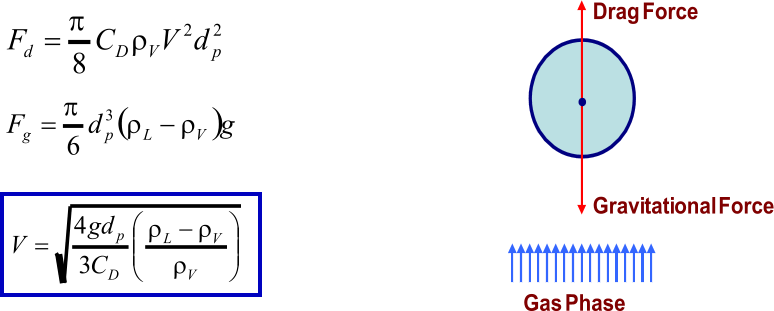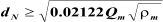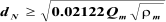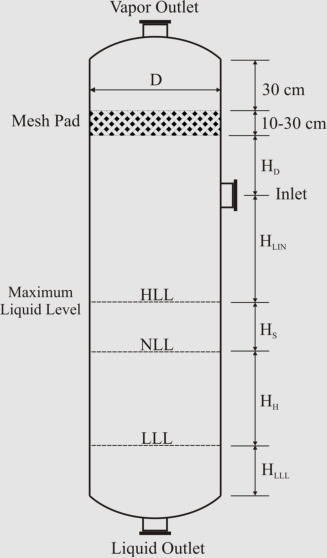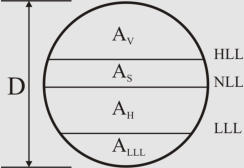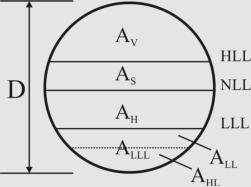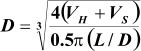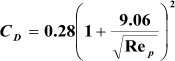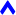Separator Sizing Here we review important guidelines and procedures for sizing multiphase separators: General Guidelines Droplet Settling Theory Liquid Retention Time Sizing Procedure Improvements Offered by CFD Simulations General Guidelines Orientation For   practical   design   of   separators,   it   is   necessary   to   compare both      horizontal      and      vertical      orientation      designs      to determine    which    design    is    more    economical    (Svrcek    and Monnery,   1993).   However,   the   following   general   guidelines are   recommended   to   be   considered   while   sizing   multiphase separators. Vertical orientation advantages : Occupy little plot space Easier    than    horizontal    separators    to    transport    and install Used    when    there    is    small    liquid    load,    limited    plot space,   or   where   ease   of   level   control   is   desired   (Evans, 1974) Preferred   for   operating   either   low   or   very   high   gas/oil ratio fluids (Arnold and Stewart, 2008) Horizontal orientation advantages : Higher operating capacity, and processing capabilities Preferred   when   emulsions,   foam,   or   high   gas/oil   ratio fluids are present (Arnold and Stewart, 2008) Separator Aspect Ratio (Length/Diameter) Plot restrictions and economic measures specify the separator aspect ratio. Different ranges have been suggested as shown in the table below Economic   studies   provided   the   separator   aspect   ratio   as   a function   of   the   operating   pressure   (Walas,   1990;   Svrcek   and Monnery,   1993).   The   recommended   values   presented   in   the table . The   figures   below   show   illustrated   guidelines   proposed   by Walas   (1990)   and   Watkins   (1967)   for   two-phase   separators with and without demisters, respectively. Design Heuristics Proposed by Walas (1990) for Two-Phase Separators Equipped with Wire Mesh Demisters. Design Heuristics Proposed by Watkins (1967) for Two- Phase Separators. There   are   also   some   general   guidelines   for   choosing   among different   horizontal   separators   (see   the   figure   below)   which are   worth   mentioning:   As   per   Arnold   and   Stewart   (2008), while    the    simple    design    is    easily    adjustable    to    handle unexpected   changes   in   liquid   density/flow   rates,   the   bucket &    weir    design    is    used    when    interface    level    control    is difficult   (emulsions   or   paraffin   problems).   If   heavy   liquid mass   fraction   is   substantial,   the   weir   design   is   preferable, but   the   boot   design   is   preferred   when   heavy   liquid   mass fraction is less than 20% (Monnery and Svrcek, 1994) . (a)                                                      (b)                                                                                                                                                                                                                                                                                 ( c ) (d)                                                                                                                         Different Common Designs of Horizontal Three-Phase Separators; (a) “Simple”, (b) “Boot”, (c) “Weir”, and (d) “Bucket and Weir”. Droplet Settling Theory Liquid Droplet in Vapor Phase The    force    balance    between    drag    and    gravitational    forces exerted    on    a    liquid    droplet    leads    to    its    terminal    settling velocit y: Drag   coefficient   for   vapor-liquid   separation   can   be   obtained from    the    diagram    proposed    by    GPSA.    This    diagram    is applicable   if   Re p    is   between   0.4   and   1500.   The   diagram   curve can   be   regressed   as   shown   in   the   following   with   SI   units (Monnery and Svrcek, 2000) : As     presented     in     the     table,     there     are     different     values recommended   by   the   field   researchers   for   the   design   droplet size . In   separator   sizing   literature,   it   is   very   common   to   rearrange the   settling   velocity   equation   introducing   a   K   coefficient, known   as   the   settling   velocity   coefficient.   This   leads   to   the Sauders-Brown equation : Different   values   are   suggested   in   the   literature   to   be   used for    the    settling    velocity    coefficient.    The    following    table provides some of these suggestions : More    sophisticated    approaches    recommend    that    pressure functionality    need    be    considered    for    the    settling    velocity coefficient.     The     approaches     proposed     by     York     Mist Eliminator   Company   and   GPSA   are   given   at   below   (Svrcek and Monnery, 1993): York Mist Eliminator Compan y GPS A Liquid Droplet in Liquid Phase Similar     to     vapor-liquid     separation,     the     force     balance between   drag   and   gravitational   forces   exerted   on   a   liquid droplet leads to its terminal velocity It   is   generally   assumed   that   Re p    is   lower   than   0.1   and   Stokes’ law can be used for calculating the drag coefficient: C D  = 24/Re p   This   assumption   results   in   a   very   simple   equation   for   the liquid droplet terminal velocity : Some   design   droplet   sizes   recommended   in   the   literature   are shown in the tabl e. Liquid Retention Time In   order   to   handle   upstream   or   downstream   variations   and establish     smooth     and     safe     operation     of     downstream facilities,     an     adequate     liquid     retention     time     need     be assumed.    This    assumption    may    be    based    on    experience, scale   model   predictions,   or   field   data.   Liquid   retention   time is     combined     from     holdup     and     surge     time.     Holdup, considered   for   smooth   and   safe   operation   of   downstream facilities,   is   defined   as   the   time   it   takes   to   reduce   the   liquid level   from   normal   to   low   liquid   level   by   closing   inlet   flow while     maintaining     a     normal     outlet     flow.     Surge     time, considered   for   handling   upstream   or   downstream   variations, is   defined   as   the   time   it   takes   to   increase   the   liquid   level from    normal    to    high    liquid    level    by    closing    outlet    flow while    maintaining    a    normal    inlet    flow.    Some    rules-of- thumb   have   been   suggested   at   literature   which   are   not   in reasonable agreement with each other as shown in the table . In   the   more   pragmatic   approach   presented   by   Svrcek   and Monnery   in   1993   and   1994,   low   liquid   level   is   first   set   based on   the   operating   pressure   and   diameter   (typical:   6-12   in). Then,   holdup   (typical:   2-10   min)   and   surge   times   (typical:   1-5 min)   are   specified   based   on   the   service   and   quality   of   the personnel   and   instrumentation   (with   impact   factor   of   1.0   1.5 altogether). Sizing Procedure The   sizing   approach   outlined   here   is   proposed   by   Svrcek and   Monnery   through   two   internationally   acclaimed   papers in   1993   and   1994.   It   is   an   algorithmic   method   for   designing the   most   economical   separator.   Among   the   classic   methods, the   method   offers   the   most   comprehensive   approach   while benefiting   from   the   accepted   industrial   design   guidelines. As   explained   in   the   following,   height   adjustments   are   made for   sizing   vertical   separators   and   iterative   calculations   are performed     for     finding     the     most     economical     horizontal separator. Sizing Vertical Two-Phase Separat or 1. Calculate the terminal settling velocity of liquid droplets. 2. Set the vapor velocity equal to 75% of the terminal settling velocity. 3. Calculate the vessel diameter based on the vapor volumetric flow-rate and the vapor velocity. 4. Obtain low liquid level height (typical: 6-12 in). 5. Calculate the height from LLL to NLL based on the required holdup time (typical: 2-10 min). 6. Calculate the height from NLL to HLL based on the surge time (typical: 1-5 min). 7. Calculate the height from HLL to the inlet nozzle centerline based on the inlet nozzle size which can be calculated as:  8. Assume the disengagement height (H D ) to be about 0.5D (Min: 42 in or 30 in with demister). 9. Calculate the vessel height. If the aspect ratio is not in the range of 1.5-6.0, increase the diameter (to decrease the aspect ratio) or increase vapor disengagement height (to increase the aspect ratio) and fix the problem. Sizing Horizontal Two-Phase Separato r In horizontal arrangement, cross-sectional area of the vessel is shared by vapor-liquid disengagement area and areas for liquid retention and low liquid level. Here is the iterative algorithmic method for sizing: 1. Calculate the terminal settling velocity of liquid droplets. Use 0.75U T as the settling velocity (25% contingency). 2. Calculate the holdup volume based on the required holdup time (typical: 2-10 min) and liquid volumetric flow rate. 3. Calculate the surge volume based on the surge time (typical: 1-5 min) and liquid volumetric flow rate. 4. Assume an aspect ratio (L/D) based on the operating pressure and calculate an initial diameter:  5. Obtain LLL (typical: 6-12 in) and calculate A LLL . 6. Set H V  to the larger of 0.2D or 1 ft (no demister), 2 ft (with demister). Calculate A V . 7. Calculate L to accommodate the liquid retention volume: L = (V H +V S )/(A T -A V -A LLL ) 8. Calculate the minimum length required for vapor-liquid separation: L min  = (Q V /A V )(H V /U T ) 9. If L < 0.8L min , increase H V  and go to step 7. Else if L < L min , set L = L min . Else if L > 1.2L min , decrease H V  (if acceptable), and go to step 7. Else (L > L min ), L is acceptable. 10. If L/D < 1.5, decrease D and go to step 5. Else if L/D > 6, increase D and go to step 5. 11. Calculate the approximate vessel weight based on the thickness and surface area of shell and heads. 12. In order to find the optimum case (the minimum weight), change the vessel diameter by 6 in increments and repeat the calculations from step 5 while keeping the aspect ratio in the range of 1.5 to 6.0. Sizing Vertical Three-Phase Separato r 1. Calculate the terminal settling velocity of light liquid droplets. 2. Set the vapor velocity equal to 75% of the terminal settling velocity. 3. Calculate the vessel diameter based on the vapor volumetric flow- rate and the vapor velocity. 4. Calculate the separation velocities of both liquid phases through each other. 5. Set the thickness of liquid phases (HLL = HHL =  0.3 m as minimum), and calculate the separation times for the liquid droplets (t HL  and t LH ).  6. Calculate the cross-sectional areas of the liquid phases. Note, A H  is the same as the vessel cross-sectional area (A H  = A), but the area allotted to baffle plate down-comer should be subtracted from A to obtain A L . 7. Calculate the residence time of the light liquid:    t RL  = H L A L /Q LL . If t RL  < t HL  increase the vessel diameter so that t RL  = t HL . 8. Calculate the residence time of the heavy liquid: t RH  = H H A H /Q HL . If t RH  < t LH  increase the vessel diameter so that t RH  = t LH . 9. Calculate the height of light liquid phase above the outlet (H R ) based on the required holdup time (typical: 2-10 min) and liquid flow rate. 10. Calculate the surge height (H S ) based on the surge time (typical: 1-5 min) and liquid flow rate. 11. Set liquid level above baffle (H A  = 0.15 m minimum). 12. Calculate the height from NLL to the inlet nozzle centerline based on the surge height (H S ) and the inlet nozzle size which can be calculated as:   13. Assume the disengagement height (H D ) to be about 0.5D (minimum: 24”+0.5d N  with demister or 36”+0.5d N  no demister). 14. Calculate the vessel height. If the aspect ratio is not in the range of 1.5-6.0, increase the diameter (to decrease the aspect ratio) or height (to increase the aspect ratio) of the separator and fix the problem. Sizing Horizontal Three-Phase Separator In     order     to     show     the     sizing     strategy,     only     “simple” horizontal      separator      is      presented      here.      The      other arrangements,    i.e.    “weir”,    “boot”,    and    “bucket    &    weir”, follow   the   same   strategy   but   require   further   effort   for   sizing their compartments. In horizontal arrangement, cross-sectional area of the vessel is shared by vapor-liquid disengagement area and areas for liquid retention and low liquid level. Here is the iterative algorithmic method for sizing: 1. Calculate the terminal settling velocity of light liquid droplets. Use 0.75U T   as the settling velocity (25% contingency). 2. Calculate the holdup volume based on the required holdup time (typical: 2-10 min) and liquid volumetric flow rate. 3. Calculate the surge volume based on the surge time (typical: 1-5 min) and liquid volumetric flow rate. 4. Assume an aspect ratio (L/D) based on the operating pressure and calculate an initial diameter:  5. Set the thickness of liquid phases (minimum: HLL = HHL = 0.3 m), and calculate A LLL  = A HL +A LL . 6. Set H V  to the larger of 0.2D or 1 ft (no demister), 2 ft (with demister). Calculate A V . 7. Calculate L to accommodate the liquid retention volume: L = (V H +V S )/(A T -A V -A LLL ) 8. Calculate the minimum length required for vapor-liquid separation: L min  = (Q V /A V )(H V /U T ) 9. If L < 0.8L min , increase H V  and go to step 7. Else if L < L min , set L = L min . Else if L > 1.2L min , decrease H V  (if acceptable), and go to step 7. Else (L > L min ), L is acceptable. 10. Calculate the liquid-liquid separation times: t HL  = (D-H V -HHL)/U HL  and t LH  = HHL/U LH 11. Calculate the light liquid residence time: t RL  = L(A-A V -A HL )/Q LL . If t RL  < t HL  increase L so that t RL  = t HL . 12. Calculate the heavy liquid residence time: t RH  = LA HL /Q HL . If t RH  < t LH  increase L so that t RH  = t LH . 13. If L/D < 1.5, decrease D (if acceptable) and go to step 5. Else if L/D > 6, increase D and go to step 5. 14. Calculate the approximate vessel weight based on thickness and surface area of shell and heads. 15. In order to find the optimum case (the minimum weight), change the vessel diameter by 6” increments, and repeat the calculations from step 5 while keeping the aspect ratio in the range of 1.5 to 6.0. Improvements Offered by CFD Simulations The    recent    research    project    by    Pourahmadi    Laleh    (2010) developed    Computational    Fluid    Dynamics    (CFD)    based simulation    of    multiphase    separators.    In    order    to    study selected   aspects   of   phase   separation,   the   focus   was   placed   on hydrocarbon-water   systems,   and   the   oilfield   separator   data ranging    from    light    oil    conditions    to    heavy    oil    conditions were    used    in    these    multiphase    separator    simulations.   An efficient   combination   of   two   multiphase   simulation   models available,      VOF      and      DPM,      with      appropriate      model assumptions   and   settings   was   used.   Two   independent   sets of   CFD   simulations,   one   for   vapor-liquid   separation   and   the other    for    liquid-liquid    separation,    were    performed    using simple and efficient grid systems. When      compared      to      classic      design      strategies,      CFD simulations    indicated    that    additional    residence    times    are necessary   for   droplets   to   pass   through   the   interfaces.   The interface    residence    time    may    be    as    high    as    around    100    s depending on the fluid properties. In    the    vapor-liquid    separation    compartment,    the    efficient droplet   size   and   the   appropriate   extra   vapor   residence   time (for     droplet     penetration     through     the     interface)     were estimated   as   a   function   of   the   vapor   density.   It   was   shown that   for   the   three-phase   separator   case   study,   the   efficient separation    of    oil    droplets    from    gas    phase    results    in    total separation of water droplets from the gas phase. For   the   liquid-liquid   separation   process,   the   upper   limit   of Stokes’   law   (Re p    <   0.1)   was   exceeded   in   several   case   studies. Hence,   Abraham   equation   (Re p    <   2000)   was   recommended   to be used instead:   Using    the    Abraham    equation    in    liquid-liquid    separation calculations,   the   efficient   droplet   size   was   estimated   based on   continuous   phase   viscosity.   Hence,   for   water   droplets,   a linear   regression   fit   based   on   the   oil   phase   viscosity   was developed.   An   oil   droplet   size   of   around   600   micron   can   be assumed   if   the   Abraham   equation   is   used   for   estimation   of the   rising   velocity   of   oil   droplets   out   of   the   water   phase. Furthermore,   it   was   shown   that   the   use   of   Stokes’   law   for interpretation   of   CFD   results   does   lead   to   a   weak   correlation between     efficient     droplet     sizes     and     continuous     phase viscosities. A      comprehensive      CFD-based      study      on      the      velocity constraints      caused      by      re-entrainment      in      horizontal separators   confirmed   that,   as   per   practical   experience,   high vapor   densities   and   high   oil   viscosities   reduce   the   maximum safe velocity of the vapor phase. The    results    of    CFD    simulations,    using    all    the    feasible horizontal      designs      and      selected      oilfield      conditions, indicated   that   the   oil   phase   does   not   re-entrain   the   water droplets,   but   the   oil   droplets   may   be   “re-entrained”   by   the water   phase   at   a   high   velocity.   It   was   also   elucidated   that   the geometry     of     water     phase     compartment     in     horizontal separators    is    a    key    factor    affecting    the    liquid-liquid    re- entrainment     phenomenon.     The     maximum     safe     cross- sectional   velocity   of   the   water   phase   was   a   linear   function   of the oil viscosity. Finally,   it   was   shown   that   the   algorithmic   design   method   of Monnery    and    Svrcek    (1994)    can    be    modified    to    use    CFD simulation   results   to   specify   a   realistic   optimum   separator design/size.      This      fact      was      elaborated      through      the mobile/desktop   applications   developed   for   sizing   two-phase and    three-phase    separators.    Please    click    here     for    more information. A   complete   and   colourful   Kindle   version   of   this   interesting PhD   thesis   can   be   downloaded   from   here    at   a   cost   of   2-3 cups of coffee! References: Abraham, F.F., “Functional Dependence of Drag Coefficient of a Sphere on Reynolds Number”, Physics of Fluids, 13, 1970, 2194-2195. Arnold, K., Stewart, M., “Surface Production Operations”, 3 rd  Edition, Elsevier, 2008. Branan, C., “The Process Engineers Pocket Handbook”, Vol. 2, Gulf, 1983. Evans, F.L., “Equipment Design Handbook for Refineries and Chemical Plants”, Vol. 2, Gulf, 1974. Gas Processors Suppliers Association, GPSA Engineering Data Book, 11 th  Edition, Vol. 1, Gas Processors Association, 1998. Gerunda, A., “How to Size Liquid-Vapor Separators”, Chemical Engineering, May 4, 1981, 81-84. Hooper, W.B., “Decantation”, Section 1.11 in “Handbook of Separation Techniques for Chemical Engineers”, Ph.A. Schweitzer (Ed.), 3 rd  Edition, McGraw-Hill, 1997. Lyons, W.C., Plisga, G.J. (Editors), “Standard Handbook of Petroleum and Natural Gas Engineering”, Volume 2, Gulf Professional Publishing, 2005. Monnery, W.D., Svrcek, W.Y., “Analytical Study of Liquid/Vapor Separation Efficiency”, PTAC, 2000. Monnery, W.D., Svrcek, W.Y., “Successfully Specify Three- Phase Separators”, Chem. Eng. Progress, September, 1994, 29-40. Pourahmadi Laleh, A., "CFD Simulation of Multiphase Separators", PhD Thesis, University of Calgary, Calgary, Canada, 2010. Pourahmadi Laleh, A., Svrcek, W.Y., Monnery, W.D., "CFD Simulation of Oilfield Separators: A Realistic Approach", LAMBERT Academic Publishing, 2011. Sinnott, R.K., “Chemical Engineering Design” in “Coulson & Richardson’s Chemical Engineering”, 2 nd  Edition, Butterworth-Heinemann, 1997. Smith, H.V., “Oil and Gas Separators”, in “Petroleum Engineering Handbook”, Bradley, H.B. (Ed), Society of Petroleum Engineers, 1987. Stokes, G.G., “On the Theories of Internal Friction of Fluids in Motion, and of the Equilibrium and Motion of Elastic Solids”, Transaction of the Cambridge Philosophical Society, 8(22), 1845, 287-305. Svrcek, W.Y. and W.D. Monnery, "Design Two-Phase Separators within the Right Limits", Chem. Eng. Prog. 89(10), 53-60, 1993. Walas, S.M., “Process Vessels”, Chapter 18 in “Chemical Process Equipment Selection and Design”, Butterworth- Heinemann, 1990. Watkins, R.N., “Sizing Separators and Accumulators”, Hydrocarbon Proc., 46(11), 1967. Wu, F.H., “Separators, Liquid-Vapor, Drum Design”, in “Encyclopedia of Chemical Processing and Design”, J.J. McKetta, W.A. Cunningham (Ed.), Marcel Dekker, 1990.